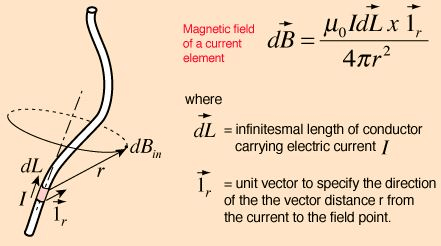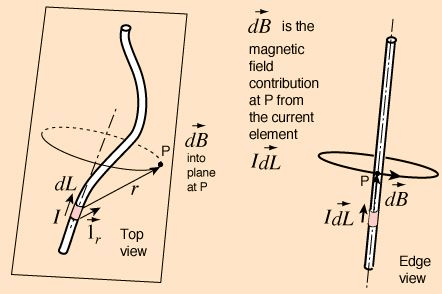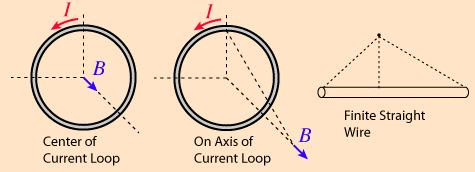Courses

# Biot-Savart's Law - Magnetism, Electromagnetic Theory, CSIR-NET Physical Sciences Physics Notes | EduRev

## Physics : Biot-Savart's Law - Magnetism, Electromagnetic Theory, CSIR-NET Physical Sciences Physics Notes | EduRev

The document Biot-Savart's Law - Magnetism, Electromagnetic Theory, CSIR-NET Physical Sciences Physics Notes | EduRev is a part of the Physics Course Physics for IIT JAM, UGC - NET, CSIR NET.
All you need of Physics at this link: Physics

Biot-Savart Law

The Biot-Savart Law relates magnetic fields to the currents which are their sources. In a similar manner, Coulomb's law relates electric fields to the point charges which are their sources. Finding the magnetic field resulting from a current distribution involves the vector product, and is inherently a calculus problem when the distance from the current to the field point is continuously changing.See the magnetic field sketched for the straight wire to see the geometry of the magnetic field of a current.

## Magnetic field contribution of a current element

Each infinitesmal current element makes a contribution to the magnetic fieldat point P which is perpendicular to the current element, and perpendicular to the radius vector from the current element to the field point P.The relationship between the magnetic field contribution and its source current element is called the Biot-Savart law.

The direction of the magnetic field contribution follows the right hand rule illustrated for a straight wire. This direction arises from the vector product nature of the dependence upon electric current.

## Biot-Savart Law Applications

Some examples of geometries where the Biot-Savart law can be used to advantage in calculating the magnetic field resulting from an electric currentdistribution.159 docs

,

,

,

,

,

,

,

,

,

,

,

,

,

,

,

,

,

,

,

,

,

,

,

,

,

,

,

;